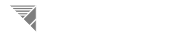The Simulator Formula Reference Page A Website For The AP Create Task

# Escape Velocity Calculation

#### The escape velocity formula is a calculation that derives the velocity needed to leave the gravitational field of a massive body to the point of no further impulse. The needed variables include the planet or moons mass in kg and radius from the planet's radius in meters. The Algorithm then deposits the velocity needed in meters per second. Some sample variable pairs are listed in the table below for user input and ranged estimations.

Mercury 3.285E23 2440
Venus 4.867E24 6052
Earth 5.972E24 6371
Mars 6.39E23 3390
Jupiter 1.898E27 69911
Saturn 5.683E26 58232
Neptune 1.024E26 24622
Uranus 8.681E25 25362

Enter the Mass(kg) of the Planet to Be Escaped In Scientific Notation (Including the 'E'): Enter the Radius(km) of the Planet to be Escaped:

# Entered Sequentialized Orbit Given Delta-V

#### In space flight, their are 3 sequences of staged orbitals beginning at sub-orbital, elliptical, and eventually hyperbolic. Then beyond the earth orbitals, a spacecraft can enter the orbits of other astronomical bodies ranging from a lunar to Jovian orbit. Based on the amount of thrust/ Delta V given by the propulsion system, a rocket will enter the orbital that coincides with the established thrust velocity. This program accepts an amount of Delta- V entered by the user and calculates the orbital that it will be intercepted, displaying each possible orbital trajectory. *NOTE The entered value must be a positive real number.

Enter the Amount of Delta-V Your Space Craft is Capable of Ommiting